# Information capacity measurements from Slanted edges: Equations and Algorithms

Current Documentation
View previous documentation
View legacy documentation
All documentation versions

 News: Imatest 23.1 (March 2023) (available in the Imatest Pilot program). New methods for calculating camera information capacity, Noise Power Spectrum (NPS), Noise Equivalent Quanta (NEQ), and Ideal observer SNR (SNRi) from slanted-edge patterns are now available.
 The basic premise of this work is that Information capacity is a superior Key Performance Indicator (KPI) of imaging systems — better than sharpness or noise, which it incorporates. Shannon information capacity can also be calculated from images of the Siemens star, introduced in 2020. Siemens Star measurements are the recommended method for calculating information capacity when artifacts from image processing (demosaicing, data compression, etc.) are of importance. Slanted-edge measurements are faster, more convenient, and better for calculating total information capacity. They work best with minimally or uniformly-processed images (without bilateral filtering) converted from raw, but the Edge Variance method also produces useful information with bilateral-filtered imaged (most JPEGs from consumer cameras).  The Siemens star method was presented at the Electronic Imaging 2020 conference, and published in the paper, “Measuring camera Shannon information capacity from a Siemens star image” and announced in the Imatest News Post: Measuring camera Shannon information capacity with a Siemens star image. The 2020 white paper, Camera information capacity: a key performance indicator for Machine Vision and Artificial Intelligence systems, is a more readable introduction to the Siemens Star measurement. This page presents new calculations of information capacity and additional measurements from slanted edges.
Photographic scientists and engineers stress the fact that no single number satisfactorily describes the ability of a photographic system to reproduce the small-scale attributes of the subject.

Nothing like a challenge! There is such a metric for electronic communication channels— one that quantifies the maximum amount of information that can be transmitted through a channel without error. The metric includes sharpness and noise (grain in film). And a camera— or any digital imaging system— is such a channel.

The metric, first published in 1948 by Claude Shannon* of Bell Labs [1,2], has become the basis of the electronic communication industry. It’s called the Shannon channel capacity or Shannon information capacity C, and has a deceptively simple equation . (See the Wikipedia page on the Shannon-Hartley theorem for more detail.)

$$\displaystyle C = W \log_2 \left(1+\frac{S}{N}\right) = W \log_2 \left(\frac{S+N}{N}\right) = \int_0^W \log_2 \left( 1 + \frac{S(f)}{N(f)} \right) df$$

W is the channel bandwidth, S(f) is the average signal energy (the square of signal voltage; proportional to MTF(f)2), and N(f) is the average noise energy (the square of the RMS noise voltage), which corresponds to grain in film. It looks simple enough (only a little more complex than E = mc2 ), but it’s not easy to apply.

*Claude Shannon was a genuine genius. The article, 10,000 Hours With Claude Shannon: How A Genius Thinks, Works, and Lives, is a great read. There are also a nice articles in The New Yorker and Scientific American. The 29-minute video “Claude Shannon – Father of the Information Age” is of particular interest to me it was produced by the UCSD Center for Memory and Recording Research. which I frequently visited in my previous career.

This page describes how to calculate information capacity C from images of slanted-edges, Imatest’s most widely-used test image, which (thanks to a recent discovery) allows signal and noise to be calculated from the same location, resulting in a superior measurement of image quality. The earlier (2020) Siemens star method is described in Shannon information capacity from Siemens stars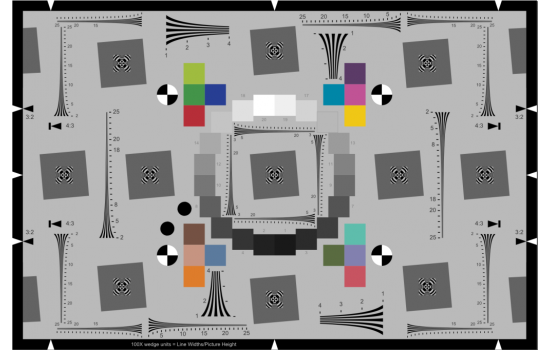The eSFR ISO chart. Automatically detected ROIs, combining MTF, color, tone, and noise measurements

## Introduction to the new measurements

This page describes several Information capacity-related measurements introduced in Imatest 23.1, released in March 2023. These measurements take advantage of newly-discovered properties of slanted edges– Imatest’s most widely used patterns for measuring MTF. Many have been used for medical imaging, but are unfamiliar elsewhere, in part because they were difficult to perform. We describe two convenient new measurement methodologies, both of which use the slanted edge.

1. The Edge variance calculation conveniently calculates camera information capacity.
2. The Noise image calculation, which uses a different approach to calculate information capacity and several additional image quality factors (NPS, NEQ, SNRi, and more).

 This page introduces the new calculations and presents detailed equations and algorithms. The Instructions page introduces the new calculations, shows how to obtain them, then presents Key Results.

Motivation —  We need to obtain information capacity from images that have very different types of image processing.

• Minimally or uniformly-processed images, converted from raw to TIFF or PNG files. By “minimally-processed”, we mean no sharpening, no noise reduction, at most a simple gamma curve (no complex tonal response curves or local tone mapping). A color matrix may be applied (it affects noise and SNR, but not MTF). When available, these images give the most reliable information capacity measurements.
• JPEG files from cameras, which usually have bilateral filters — filters that sharpen images near contrasty features like edges but blur them to reduce noise elsewhere — making it appear that the image contains more information than it actually has. This improves conventional SNR measurements (made from flat patches) while actually removing information.

In late October 2022, we discovered how to extract signal-dependent noise from slanted-edges using an overlooked capability of the ISO 12233 slanted-edge algorithm, described briefly below and in more detail in the white paper, Measuring Camera Information Capacity from Slanted-edges

In March 2023, we discovered a second overlooked capability that allows Imatest to calculate a camera’s Noise Power Spectrum (NPS), Noise Equivalent Quanta (NEQ), and Ideal Observer SNR (SNRi), but only works with minimally / uniformly-processed images.

The slanted-edge method of calculating MTF, which has been part of the ISO 12233 standard since 2000,

• takes each scan line y(x) in a slanted-edge Region of Interest (ROI),
• finds its center,
• fits a polynomial curve to the centers, then
• depending on the relation between the line center and the curve, adds the line contents to one of four bins.
• The bins are then interleaved, resulting in a 4× oversampled averaged edge, which has lower noise than the individual scan lines.
• Calculates MTF (Modulation Transfer Function, usually synonymous with Spatial Frequency Response), by differentiating the averaged edge to obtain the Line Spread Function, LSF, windowing the LSF, then Fourier-transforming it. MTF is the absolute value of the Fourier Transform normalized to 1 (or 100%) at zero frequency.

The new information capacity measurements take advantage of overlooked capabilities of the slanted-edge method.

#### Why two calculations (Edge variance and Noise image)?

The Edge variance was developed first, starting in late October 2022. It was presented at the Electronic Imaging 2022 conference. The Noise image was developed in February 2023, about a month after the conference. It measures more image quality parameters than the Edge variance method.

We currently recommend the Noise image method because we believe the camera information capacity measurement is slightly more accurate. The reason: it calculates the Noise Power Spectrum, while the Edge variance assumes the NPS is flat (white). On the other hand, The Edge variance method can provide useful (if imperfect) results for bilateral-filtered images (most JPEGs from consumer cameras), while the Noise image method is only recommended for uniformly or minimally-processed images.

### A: Edge variance calculation

Summary:  Sum the squares of the scan lines to obtain the edge variance, then use it to calculate information capacity.

The Edge variance Information capacity calculation is described in detail in the white paper, New Slanted-Edge Image Quality Measurements: the Edge variance calculation, and in the paper presented at Electronic Imaging 2023, “Measuring Camera Information Capacity with Slanted Edges. The concise descriptions on this page  omit many of the details in the two linked documents.

The calculation starts with images of slanted edges, (Original ROIbelow), typically made from a 4:1 contrast chart (chart contrasts between 2:1 and 10:1 are acceptable). In addition to the binning/summing described above, the squares of the scan lines are summed. This allows the variance of the edge, σs2(x), which is equivalent to the signal-dependent noise power, N(x), to be calculated.

$$\displaystyle \sigma_s^2(x) = \frac{1}{L} \sum_{l=0}^{L-1} (y_l(x)-\mu_s(x))^2 = \frac{1}{L}\sum_{l=0}^{L-1} y_l^2(x) \ – \left(\frac{1}{L}\sum_{L=0}^{L-1} y_l(x) \right)^2$$

Noise power for the Shannon-Hartley Equation  N(x) = σs2(x) and voltage σs(x) are important because many images— including most JPEGs from consumer cameras— have bilateral filters, which sharpen the image (boosting noise) near sharp areas like edges, and blurs it (to reduce visible noise) elsewhere. This obscures the noise at edges, which is critical to the performance — and information capacity — of the system. The new technique makes signal-dependent noise near the edge visible so that it can be used in the information capacity calculation. It is also highly convenient.

The selection of N depends on the image processing. Two major classes have been identified.

1. Uniformly or minimally-processed images, often TIFFs converted from raw files (raw→TIFF) without bilateral filtering, i.e., they either have no or uniform sharpening or noise reduction. Most cameras intended for Machine Vision/Artificial Intelligence fall into this category.

Since noise can be a very rough function of x, a large region size is required for a stable value of N. We average over all values of x in the ROI.
$$N_{uniform} = \text{mean}(\sigma_s^2(x))$$ for all values of x in the ROI.

2. Bilateral-filtered images include most JPEG images from consumer cameras.

Bilateral filters sharpen images near contrasty features such as edges, but blur them (to reduce noise) elsewhere. This causes a noise peak near the edge (below, left). The blurring improves Signal-to-Noise Ratio (SNR), but it removes information. Because of this, noise near the edge can dominate camera performance, and should be strongly weighted in calculating N. We have long known about the noise peak, but until the present method was developed, there was no easy way to observe or measure it (or detect bilateral filtering).

For calculating information capacity C, we use the square of the voltage, σ, at the peak, smoothed (with a rectangular kernel of length PW20/2) to remove jaggedness. $$N_{bilateral} = \sigma^2_{peak}$$. This is a somewhat arbitrary choice that produces reasonably consis­tent results. This method also works with uniformly-processed images, but results are less consistent.

Imatest lets you select the calculation of noise N: it can be Nuniform, Nbilateral , or automatically detected depending on the presence of a peak.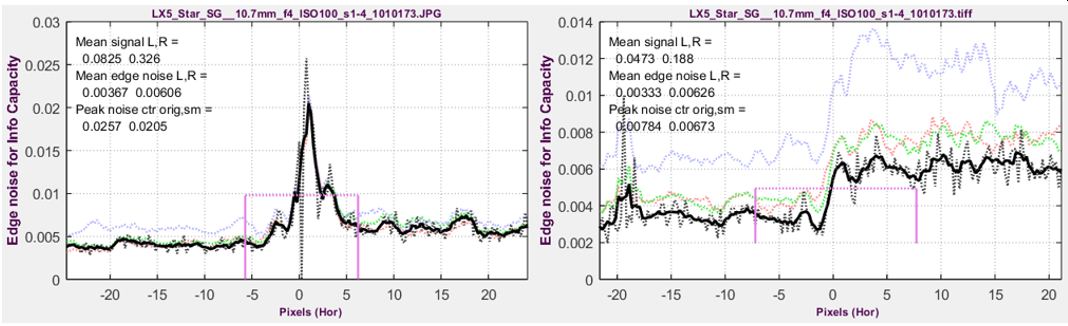Edge noise voltage for compact camera @ ISO 100.  Left: Bilateral-filtered in-camera JPEG;  Right Unsharpened TIFF from raw.
The x-axis is the original pixel location of the 4× oversampled signal.

Signal power for the Shannon-Hartley Equation  The mean signal amplitude for a uniformly-distributed signal of peak-to-peak amplitude VP-P is $$V_{avg}(f) = V_{P-P} MTF(f) / \sqrt{12}$$ — a reasonable number to use for the information capacity calculation using the Shannon-Hartley equation, shown above, which actually uses the average signal power, $$S_{avg}(f) = V^2_{P-P} MTF(f)^2 / 12$$.

After removing newly-discovered binning noise, selecting noise power calculation, and adjusting the signal level from the edge, which is a square wave, to be more representative of an “average’ signal, numbers are entered into the Shannon-Hartley equation (above) to calculate the information capacity, for the chart contrast.

Bandwidth W  is always 0.5 cycles/pixel (the Nyquist frequency). Signals above Nyquist do not contribute to the information content; they can reduce it by causing aliasing — spurious low frequency signals like Moiré that can interfere with the true image. Frequency-dependence comes from MTF(f).

Savg(f), N, and W are entered into the Shannon-Hartley equation to obtain information capacity C.

$$\displaystyle C = \int^{0.5}_0{\log_2\left(1+\frac{S_{avg}(f)}{N}\right)}df \ \approx \sum_{i=0}^{0.5/\Delta f} {\log_2\left(1+\frac{S_{avg}(i\Delta f)}{N}\right)} \Delta f$$

The key results are

C4 is the direct result of measuring 4:1 contrast ratio slanted-edges. It is calculated from the Shannon Hartley equation, using several assumptions (that the signal is uniformly distributed over the peak-to-peak measurement and the noise power spectral density (NPD) is flat). C4 is a special case of Cn, for a n:1 contrast ratio (with ISO standard 4:1 strongly recommended) Cn is sensitive to chart contrast ratio and exposure, making it interesting for measuring performance as a function of exposure but less robust than ideal for calculating a camera’s maximum information capacity.

Cmax is a much more stable measurement of the maximum information capacity for the camera starting with C4 (for the 4:1 contrast chart). It is also insensitive to exposure, at least for linear sensors, where noise is a known function of signal voltage.

Here are some key results of the Edge variance method.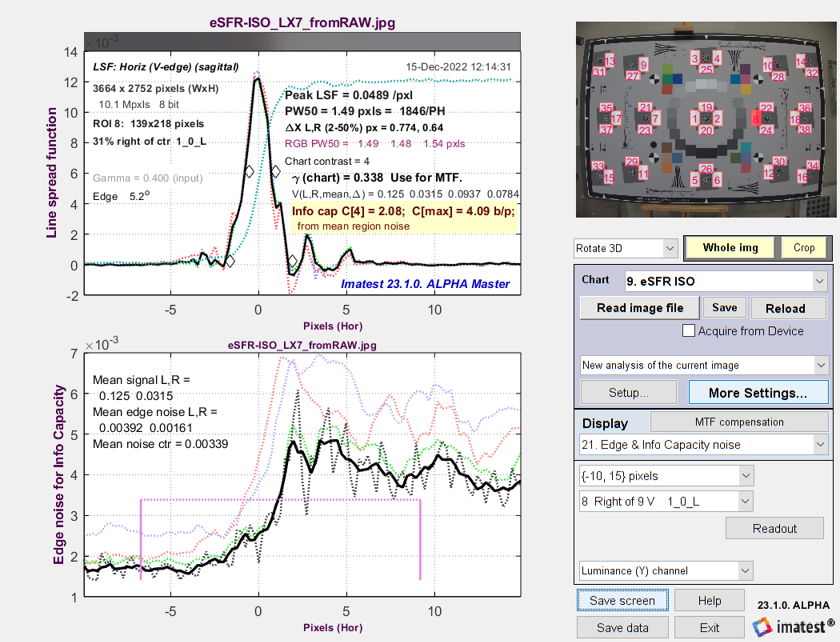Line Spread Function (LSF) and signal-dependent noise σ from
eSFR ISO image converted from raw with minimal processing

### B. Noise image calculation

Summary: Subtract a low-noise reverse-projected / de-binned ROI image from the original image to obtain a noise image, which can be used to calculate Noise Power Spectrum (NPS) and several additional measurements.

 Measurement Description Noise Power Spectrum, NPS(f) NPS was implicitly assumed to be constant (white noise) in the Edge variance method. Noise Equivalent Quanta, NEQ(f) and NEQinfo(f) measures of frequency-dependent signal-to-noise ratio (SNR). $$NEQ(f) = \mu^2\ MTF^2(f) / NPS(f)\text{, where }\mu = V_{mean}$$ has been used for quantifying medical image quality, but are much less familiar in general imaging. NEQ(f) is equivalent to the number of quanta detected by the sensor when photon shot noise is dominant. It is appropriate for calculating Digital Quantum Efficiency (DQE), when the density of quanta reaching the image sensor is known. NEQinfo(f) is derived from $$\mu = V_{P-P} / \sqrt{12}$$, making it well-suited for calculating information capacity CNEQ. Information capacities C4-NEQ and Cmax-NEQ correspond to C4 and Cmax from the Edge variance method, but are derived from NEQinfo(f). They are close, but not identical. Ideal observer Signal-to-Noise Ratio, SNRi From Skorka and Kane , “The Ideal Observer is a Bayesian decision maker that maximizes the statistical precision of a hypothesis test with two possible outcomes.” SNRi as we present is, is a metric of the detectability of small objects (squares or rectangles), typically of low contrast. The inverse Fourier transform of the Noise Voltage Spectrum. Related to sensor electrical crosstalk.

This method involves inverting the ISO 12233 binning procedure. Noting that the 4× oversampled edge was created by interleaving the contents of 4 bins, we apply an inverse of the binning algorithm to set the contents of each scan line to its corresponding bin (Inverse binned… ROI, below). Since the inverse-binned image is nearly noiseless, we can create a noise image by subtracting the inverse-binned image from the original image. This image is shown, adjusted to make the mean (zero) value middle gray, as the Noise image ROI, below

The 4× oversampled averaged edge, described above, was created by adding each scan line in the original ROI image (below, left) to one of four interleaved bins, each of which contains an averaged (noise-reduced) signal. It can be de-interleaved (de-binned or reverse-projected; the nomenclature isn’t final) by filling each line in a new image with the averaged signal of the corresponding interleave. This creates a low-noise replica of the original image (below-middle).

A noise image can be created by subtracting the reverse-projected image from the original image, correct for nonuniformity along the direction of the edge. The three images are shown below. The noise image (below-right), which has a mean value of 0, has been lightened and contrast-boosted for display. The three images are linear: a gamma curve has been applied for display.

These images allow several key image quality parameters to be calculated, including Noise Power Spectrum and Noise Equivalent Quanta, well-known in medical imaging systems, and described in an excellent review paper by Ian Cunningham and Rodney Shaw . These measurements are not well-known outside of medical imaging, largely because they have been difficult to measure.

####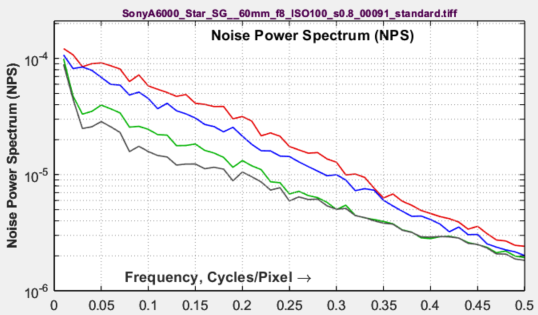Noise Power Spectrum (NPS)

(the square of the Noise (voltage) spectrum) is calculated by taking the 2D Fourier transform of the noise ROI (Region of Interest) and noting that the initial 2D spectrum has zero frequency at the center of the image. A 1D Noise Power Spectrum, NPS1, is calculated by dividing the 2D spectrum into several annular regions (the number depends on the size of the ROI), then taking the average noise power of each region. This transformation allows calculations to be performed in one dimension (rather than two) under the assumption that the vertical and horizontal MTFs are close.

The relationship between NPS and the variance of the noise image is given in equations (3) and (8) of Cunningham and Shaw , which we have reduced to one dimension and with the integration limits changed from {-∞,∞} to {0, fNyq}, where fNyq = Nyquist frequency = 0.5 cycles/pixel.

$$\displaystyle \sigma^2 = \int_0^{f_{Nyq}} NPS(f) df$$

The 1D Fourier transform described above must be scaled to be consistent with the above equation.

$$\displaystyle NPS(f) = \frac{NPS_1(f)\ \sigma^2}\int_0^{f_{Nyq}} NPS_1(f) df$$

####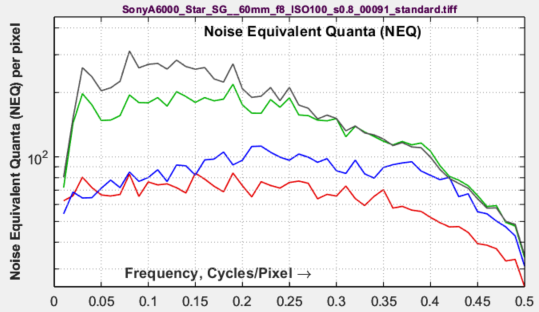Noise Equivalent Quanta (NEQ)

is a well-known figure of merit in medical imaging, but is unfamiliar in general imaging. It is described in a 2016 paper by Brian Keelan . Essentially, it is a frequency-dependent Signal-to-Noise (power) Ratio. Units are the equivalent number of quanta that would generate the measured SNR when photon shot noise is dominant.

$$\displaystyle NEQ(f) = \frac{\mu^2 MTF^2(f)}{NPS(f)}$$

where the mean linear signal, μ, can be defined in either of two ways, depending on how NEQ is to be interpreted.

If NEQ is to be used for calculating DQE (Digital Quantum Efficiency), where $$DQE(f) = NEQ(f) / \overline{q}$$, then  μ should be the mean value of the linearized signal voltage in the original image. Measuring DQE requires a separate measurement of the mean number of quanta reaching each pixel. We may add this in the future.

Getting familiar with the meaning and use of NEQ will take some time. Characterization of imaging performance in differential phase contrast CT compared with the conventional CT: Spectrum of noise equivalent quanta NEQ(k) by Tang et. al. is an excellent example of how NEQ is used in medical imaging: it has real technical depth.

####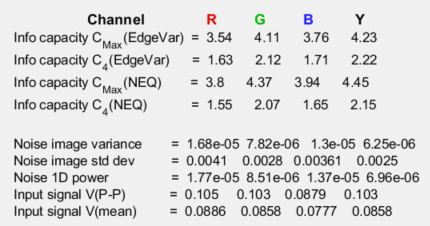Information capacity from NEQ:

A special form of NEQ, NEQinfo(f), calculated using $$\mu = V_{P-P}/\sqrt{12}$$, is used to calculate information capacity, CNEQ, from a special case of the Shannon-Hartley equation. NEQinfo is not plotted.

$$\displaystyle C_{NEQ} = \int_0^W \log_2 \left( 1 + NEQ_{info}(f)\right) df$$

where bandwidth W is the camera’s Nyquist frequency, $$W = f_{Nyq} = 0.5 \text{ Cycles/Pixel}$$. [Author’s note: I thought I’d discovered this connection, but it’s in papers on PET scanners and Digital Mammography by Christos Michail et. al. [6,7] Not papers anybody outside medical imaging is like to chance upon.]

#### Ideal Observer SNR (SNRi)

is a measure of the detectability of small objects. It is described in papers by Paul Kane  and Orit Skorka and Paul Kane . There is a problem with the equations for SNRi in these two papers. They are presented in both one and two dimensions, even though the Fourier transform of the object to be detected is expressed in two dimensions.

In , the equation is presented in one dimension,

$$\displaystyle SNRi^2 = \int_0^{f_{Nyq}}{\frac{|G(\nu)|^2 MTF^2(\nu)}{NPS(\nu)} }d\nu$$

where spatial frequency ν has units of Cycles/Pixel, and the linearized signal is normalized to have a maximum value of 1.

In , it is presented in two dimensions.

$$\displaystyle SNRi^2 = \int \int \left( \frac{\mu^2 \Delta S^2(\nu_x,\nu_y) MTF^2(\nu_x,\nu_y) }{NPS(\nu_x,\nu_y)} \right) d\nu_xd\nu_y$$

We assume that G(ν)2 is the same as μ2ΔS2(νx,vy) and that $$\nu_x = \nu_y = \nu$$, which makes MTF and NPS  essentially one-dimensional

The object to be detected is typically a rectangle of dimensions w × kw, where k = 1 (for a square) or 4 for a 1×4 aspect ratio rectangle. Its amplitude (for now) is the peak-to-peak voltage of the slanted edge (shown in the Voltage statistics figure, above), $$\Delta Q = V_{P-P}$$ which typically has a 4:1 contrast ratio.

$$\displaystyle \Delta g(x,y) = \Delta Q \cdot \text{rect}(x/w) \cdot \text{rect}(y/kw) \ , \ \text{ where rect}(x) = 1 \text{ for } -1/2 < x < 1/2 \text{ ; 0 otherwise.}$$

G(υ) is the Fourier transform of the object to be detected, Δg(x,y). It can be expressed in one or two dimensions.

In two dimensions, $$\displaystyle G_{2D}(\nu_x,\nu_y) = kw^2 \Delta Q \frac{\sin(\pi w \nu_x)}{\pi w \nu_x} \frac{\sin(\pi kw \nu_y)}{\pi k w \nu_y}$$ ,   where, as we noted,   $$\nu_x = \nu_y = \nu$$

In one dimension,  $$\displaystyle G_{1D}(k \nu) = kw \Delta Q \frac{\sin(\pi kw \nu)}{\pi k w \nu}$$

To deal with ambiguity about how to evaluate the integral, we assume that the correct equation is the double integral, where the inner part is evaluated first, then the outer part.

$$\displaystyle SNRi^2 = \int \left[ \int \left( \frac{G_{1D}(\nu)^2 MTF^2(\nu) }{NPS(\nu)} \right) d\nu \right] \left( \frac{G_{1D}(k \nu)^2 MTF^2(\nu) }{NPS(\nu)} \right) d \nu$$

We choose this in preference to single integral we previously tried.  $$\displaystyle SNRi^2 = \int \left( \frac{G_{2D}(\nu)^2 MTF^2(\nu) }{NPS(\nu)} \right) d\nu$$1D SNRi dB.  Note that this resembles the 2D plot (above), but the values (y-axis) are half of 2D SNRi.

1D analysis — Because of the conflicts and ambiguities of 2D analysis, we have also considered the simpler 1D analysis.

$$\displaystyle SNRi^2 = \int \left( \frac{G_{1D}(\nu)^2 MTF^2(\nu) }{NPS(\nu)} \right) d\nu$$

As of March 2023, we are working to resolve the question of which equation is best. The good news is that they correlate well with each other. They differ mostly in absolute magnitude.

SNRi is displayed for each color channel for w from 1 to 40 in increments of approximately the square root of w (1, 1.4, 2, …). The images below are for squares with widths w = 1, 2, 3, 4, 7, 10, 14, 20.

#### Object visibility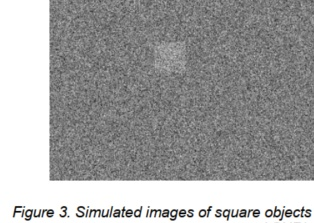Predicting object visibility for small, low contrast squares or 4:1 rectangles is the goal of SNRi measurements. The SNRi prediction begs for visual confirmation. A simulated image that can do this is shown in Figure 3 of a classic SNRi paper . We have developed a display for Imatest that does this with a real slanted-edge image and a bit of smoke and mirrors. Despite the trickery, the data is directly from the acquired image. We show two sets of results: one for a relatively low noise image and one high noise image (both from a camera with 1 inch sensors, at ISO 200 and 12800, respectively. The sides of the squares are w = 1, 2, 3, 4, 7, 10, 14, and 20 pixels. The original chart had 4:1 contrast ratio (light/dark = 4), equivalent to a Michelson contrast CMich ((light-dark)/(light+dark)) of 0.6. The outer squares have CMich = 0.6. The middle and inner squares have CMich = 0.3 and 0.15, respectively. How to use these images — Inconspicuous magenta bars near the margins are designed to help finding the small squares, which are hard to see. The SNRi curves are (initially, at least) for the chart contrast — with 4:1 (the ISO 12233 standard) strongly recommended. The outer patches correspond to the SNRi curves, and according to the Rose theory, SNRi of 5 (14 dB) should correspond to the threshold of visibility.Low noise image, ISO 200SNRi curve for the noisy image, above-right Only original pixels were used in these two images, but we used a little smoke and mirrors to make the squares with borders that have the same blur as the device under test. The SNRi curve on the right is for the noisy ISO 12800 image on the right, above. The w = 1 squares are not visible at all; the w = 2 squares are marginally visible, and w = 3 is clearly visible. In the plot, SNRi at w = 2 is 8-12 dB; it’s 11-18 dB for w =3; not too far from expectations. How the squares were made Expand the image if needed (if the original is less than 170 pixels wide) to make room for all the squares by adding mirrored versions of image to the left and right to the sides of the image. If needed, add a cropped vertical mirrored image to the bottom.  Create a (horizontal) mirror of the full image. This is the “mirror” part. Create a mask with the shares. The background is 0 and the squares are 1. The sides are sharp. Blur the squares with the MATLAB filter2 function. This is the “smoke” part. Determining the blur kernel was challenging. We found that we couldn’t get good results by just taking the 1D Line Spread function (LSF) and using it in 2D. A more complex transformation was required.  Linearize the two images (remove the gamma encoding). Combine the them using the mask, keeping the original image where the mask = 0, using the mirrored image where the mask = 1, and blending them elsewhere. Reapply the gamma encoding.

#### Noise autocorrelation

This plot is still in the R&D phase. The author (NLK) added it to examine his hypothesis that the noise power spectrum (and autocorrelation) indicate the amount of electrical crosstalk of image sensor when the effects of demosaicing and fixed-pattern noise are removed (not the case for the image on the right) and the primary noise source is photon shot noise. The idea behind the hypothesis is that light incident on the sensor is entirely uncorrelated, so that if there were no crosstalk the noise would be white.

This image on the right was white-balanced.

The curve is the inverse Fourier transform of the noise spectrum, based on the author’s limited understanding of the Wiener-Khinchin theorem.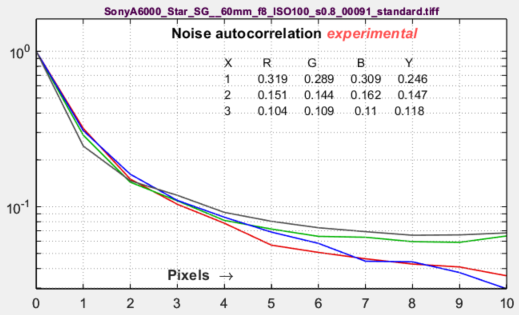The image on the right is not White-Balanced. The red channel has a larger autocorrelation distance than the other channels, as we would expect.  Click on the image to enlarge it.

A similar autocorrelation plot can also be obtained from a flat field image in the Image Statics module. The relatively large autocorrelation (>1.3) at large distances (>4 pixels) is a definite concern.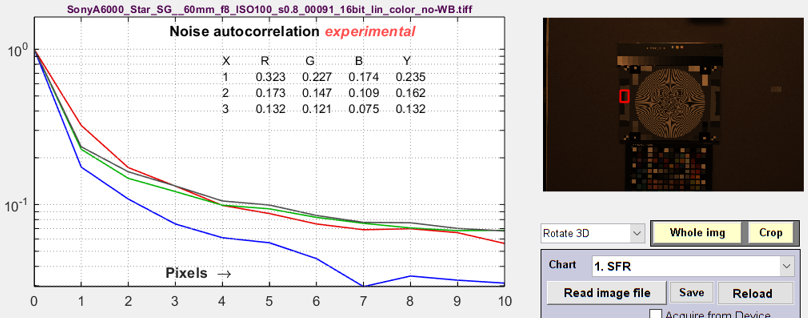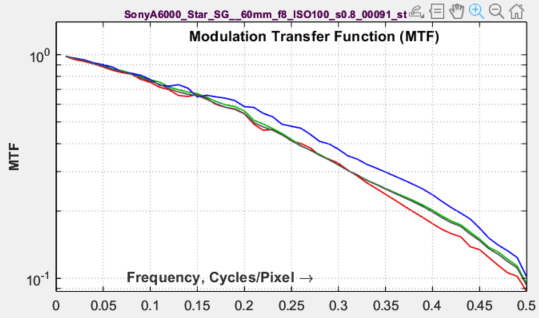Modulation Transfer Function (MTF) — looks a little different from the standard MTF plot because the y-axis is logarithmic.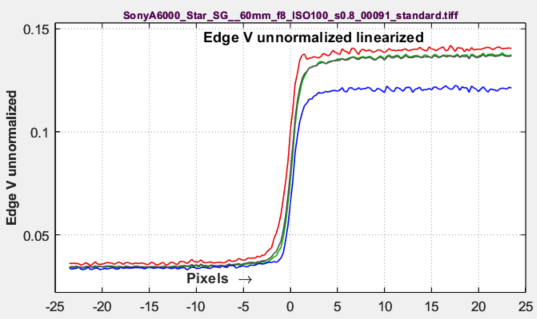Edge voltage unnormalized — used as input for the NEQ calculation. Interesting to compare peak-to-peak amplitudes of the different channels.

## Meaning of Shannon information capacity

(The Appendix in the white paper, Measuring Camera Information Capacity with slanted-edges,
has a concise definition of information.)

In electronic communication channels the information capacity is the maximum amount of information that can pass through a channel without error, i.e., it is a measure of channel “goodness.” The actual amount of information depends on the code— how information is represented. But although coding is integral to data compression (how an image is stored in a file), it is not relevant to digital cameras. What is important is the following hypothesis:

Hypothesis: Perceived image quality (assuming a well-tuned image processing pipeline) and also the performance of machine vision and Artificial Intelligence (AI) systems, is proportional to a camera’s information capacity, which is a function of MTF (sharpness), noise, and artifacts arising from demosaicing, clipping (if present), and data compression.

I stress that this statement is a hypothesis— a fancy mathematical term for a conjecture. It agrees with my experience and with numerous measurements, but it needs more testing and verification.  Now that information capacity can be conveniently calculated with Imatest, we have an opportunity to learn more about it.

The information capacity, as we mentioned, is a function of both bandwidth W and signal-to-noise ratio, S/N

 In texts that introduce the Shannon capacity, bandwidth W is often assumed to be the half-power frequency, which is closely related to MTF50. Strictly speaking, W log2(1+S/N) is only correct for white noise (which has a flat spectrum) and a simple low pass filter (LPF). But digital cameras have varying amounts of sharpening, which can result in response curves with response that deviate substantially from simple LPF response. For this reason we use the integral form of the Shannon-Hartley equation: $$\displaystyle C = \int_0^W \log_2 \left( 1 + \frac{S(f)}{N(f)} \right) df = \int_0^W \log_2 \left(\frac{S(f)+N(f)}{N(f)} \right) df$$ S and N are mean values of signal and noise power; they are not directly tied to the camera’s dynamic range (the maximum available signal). For this reason, we reference calculations of C to the contrast ratio of the chart used for the measurement, most frequently C4 for 4:1 contrast charts that conform to the ISO 12233 standard. For Siemens star analysis, we this equation was altered to account for the two-dimensional nature of pixels by converting it to a double integral, then to polar form, than back to one dimension. But this wasn’t necessary for slanted-edges, which are already one dimensional.

The beauty of both the Siemens Star and Slanted-edge methods is that signal power S and noise power N are calculated from the same location: important because noise is not generally constant over the image.

## Summary

• Shannon information capacity C has long been used as a measure of the goodness of electronic communication channels. It specifies the maximum rate at which data can be transmitted without error if an appropriate code is used (it took nearly a half-century to find codes that approached the Shannon capacity). Coding is not an issue with imaging. Rodney Shaw’s paper from 1962  is a particularly good example of measuring C for photographic film— it wasn’t easy back then.
• C is ordinarily measured in bits per pixel. The total capacity is $$C_{total} = C \times \text{number of pixels}$$.
• The channel must be linearized before C is calculated, i.e., an appropriate gamma correction (signal = pixel level gamma, where gamma ~= 2 for images in standard color spaces such as sRGB or Adobe RGB) must be applied to obtain correct values of S and N. The value of gamma (close to 2) can be determined from runs of any of the Imatest modules that analyze grayscale step charts: StepchartColorcheck., Color/ToneMultitestSFRplus, or eSFR ISO. But in most cases it can be determined from the edge image if the chart contrast is entered and Use for MTF is checked.
• We hypothesize that C can be used as a figure of merit for evaluating camera quality, especially for machine vision and Artificial Intelligence cameras. (It doesn’t directly translate to consumer camera appearance because they have to be carefully tuned to reach their potential, i.e., to make pleasing images). It provides a fair basis for comparing cameras, especially when used with images converted from raw with minimal processing.
• Imatest calculates the Shannon capacity C for the Y (luminance; 0.212*R + 0.716*G + 0.072*B) channel of digital images, which approximates the eye’s sensitivity. It also calculates C for the individual R, G, and B channels as well as the Cb and Cr chroma channels (from YCbCr).
• Shannon capacity has not been used to characterize photographic images because it was difficult to calculate and interpret. But now it can be calculated easily, its relationship to photographic image quality is open for study.
• Since C is a new measurement, we are interested in working with companies or academic institutions who can verify its suitability for Artificial Intelligence systems.
 Note: A slanted-edge information capacity measurement used prior to Imatest 2020, used primarily to obtain total information capacity from Siemens star measurements, has been deprecated completely because it was not sufficiently accurate.

1. C. E. Shannon, “A mathematical theory of communication,” Bell Syst. Tech. J., vol. 27, pp. 379–423, July 1948; vol. 27, pp.
623–656, Oct. 1948.
2. C. E. Shannon, “Communication in the Presence of Noise”, Proceedings of the I.R.E., January 1949, pp. 10-21.
3. Wikipedia – Shannon Hartley theorem  has a frequency dependent integral form of Shannon’s equation that is applied to both Imatest’s sine pattern and slanted edge Shannon information capacity calculation.
4. Brian W. Keelan, “Imaging Applications of Noise Equivalent Quanta”  in Proc. IS&T Int’l. Symp. on Electronic Imaging: Image Quality and System Performance XIII,  2016,  https://doi.org/10.2352/ISSN.2470-1173.2016.13.IQSP-213.
5. Michail C, Karpetas G, Kalyvas N, Valais I, Kandarakis I, Agavanakis K, Panayiotakis G, Fountos G., Information Capacity of Positron Emission Tomography ScannersCrystals. 2018; 8(12):459. https://doi.org/10.3390/cryst8120459
6. Christos M. Michail, Nektarios E. Kalyvas, Ioannis G. Valais, Ioannis P. Fudos, George P. Fountos, Nikos Dimitropoulos, Grigorios Koulouras, Dionisis Kandris, Maria Samarakou, Ioannis S. Kandarakis, “Figure of Image Quality and Information Capacity in Digital Mammography”, BioMed Research International, vol. 2014, Article ID 634856, 11 pages, 2014. https://doi.org/10.1155/2014/634856
7. Paul J. Kane, “Signal detection theory and automotive ima­ging”, Proc. IS&T Int’l. Symp. on Electronic Imaging: Autonomous Vehicles and Machines Conference,  2019,  pp 27-1 – 27-8,  https://doi.org/10.2352/ISSN.2470-1173.2019.15.AVM-027.
8. Orit Skorka, Paul J. Kane, “Object Detection Using an Ideal Observer Model”,  IS&T Int’l. Symp. on Electronic Imaging: Autonomous Vehicles and Machines,  2020,  pp 41-1 – 41-7, https://doi.org/10.2352/ISSN.2470-1173.2020.16.AVM-041.
9. R. Shaw, “The Application of Fourier Techniques and Information Theory to the Assessment of Photographic Image Quality”, Photographic Science and Engineering, Vol. 6, No. 5, Sept.-Oct. 1962, pp.281-286. Reprinted in “Selected Readings in Image Evaluation,” edited by Rodney Shaw, SPSE (now SPIE), 1976. A fascinating and difficult calculation of information capacity of photographic film. Available for download
10. X. Tang, Y. Yang, S. Tang, Characterization of imaging performance in differential phase contrast CT compared with the conventional CT: Spectrum of noise equivalent quanta NEQ(k), 2012 Jul; 39(7): 4467–4482. Published online 2012 Jun 29. doi: 10.1118/1.4730287.

#### Appendix 1. Binning noise

Binning noise, which has identical statistics to quantization noise, is a recently-discovered artifact of the ISO 12233 binning algorithm. It is largest near the image transition — where the Line Spread Function $$LSF(x) = d\mu_s(x)/dx$$ is maximum, and it can affect information capacity measurements. It appears because the individual scan lines are added to one of four bins, based on a polynomial fit to the center locations of the scan lines, which is a continuous function.

Assume that n identical signals μs(x) are binned over an interval {-Δ/2, Δ/2}, where Δ = 1 in the 4× oversampled output of the binning algorithm (noting that Δ = (original pixel spacing)/4). If there were no binning noise, we would expect the binning noise power σBnoise2 to be zero. However, the values of μs(xk) are summed at uniformly-distributed locations xk over the interval Δ, so they take on values

$$\displaystyle \mu_k = \mu_s(x_k) = \mu_s(x_0+\delta) = \mu_s(x_0) + \delta\ \frac{d\mu(x)}{dx} = \mu_s(x_0) + \delta\ LSF(x)$$

for Line Spread Function LSF(x). Noting that δ is uniformly distributed over {-1/2, 1/2} we apply the equation for the variance of a uniform distribution (similar to  quantization noise) to get

$$\sigma_{Bnoise}^2(x) = LSF^2(x)\ \sigma^2_{Uniform} = LSF^2(x)/12 \ \ \ \ \text{ or }\ \ \ \ \sigma_{Bnoise} = LSF(x)/\sqrt{12}$$.

Although this equation involves some approximations, we have had good success calculating the corrected noise, $$\sigma_s^2(\text{corrected}) = \sigma_s^2 – \sigma^2_{Bnoise}$$. Binning noise has no effect on conventional MTF calculations.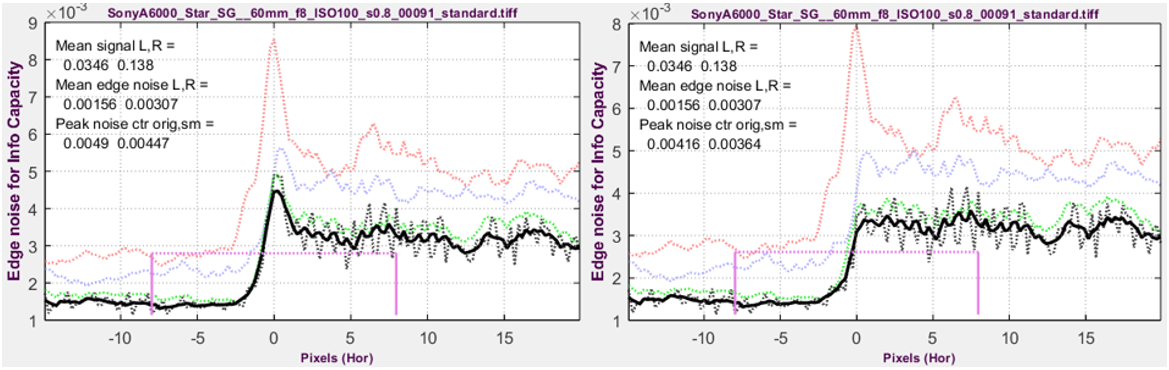Edge with binning noise                                     Binning noise removed
from a raw image from a Micro Four-Thirds camera, ISO 100, converted to TIFF with minimal processing

Binning noise also affects JPEG files with bilateral filtering (nonuniform sharpening). Removing it is important for robust calculations.

#### Appendix 2. SNRi information capacity —  We are not pursuing it because it doesn’t appear to offer sufficient new information.

Here is a bit of pure R&D speculation.  I don’t like the SNRi equation because SNRi keeps increasing for large feature widths, w, and I can’t discern any meaning for the increase. I’m writing this to get feedback on the new measurement. If it’s negative, I’ll remove it.

Using a one-dimensional (1D) analysis and equations (3) and (13) from ,

$$\displaystyle SNRi^2 = \int_0^{f_{Nyq}}{\frac{|G_{1D}(\nu)|^2 MTF^2(\nu)}{NPS(\nu)} }d\nu$$  where, in one dimension,

$$\displaystyle G_{1D}(\nu) = w \ \Delta Q \frac{\sin(\pi w \nu)}{\pi w \nu} = \frac{\Delta Q \sin(\pi w \nu)}{\pi \nu}$$

where w is the width of the rectangle, k is the aspect ratio, $$\Delta Q = V_{P-P}$$ (as defined above), and  ν = f = frequency, and fNyq = 0.5  cycles/pixel. Because the integrand has the form, S(f)/N(f), similar to the contents of the Shannon-Hartley Equation, we define a SNRi information capacity, CSNi (shortened so it rolls off the tongue more easily) in the equation below.

$$\displaystyle C_{SNi} = \int_0^{f_{Nyq}}\log_2 \left(1 + {\frac{|G_{1D}(\nu)|^2 MTF^2(\nu)}{NPS(\nu)} } \right) d\nu$$

Here are two examples. The code is temporary; not yet in any release. The y-axis label for CSNi (below right) is not correct.

Note that CSNi  appears to approach a constant value for large w, and the equation for CSNi uses G(ν)2. The absolute value bars (|…|) are redundant.

$$\displaystyle G(\nu)^2 =\frac{ \Delta Q^2 \sin^2(\pi w \nu) }{(\pi \nu)^2}$$    where   $$\sin^2(x) = (1-\cos(2x))/2$$.

$$\displaystyle G(\nu)^2 =\frac{ \Delta Q^2 (1-\cos(2\pi w \nu)) }{2 (\pi \nu)^2}$$

Note that in the limit as w → ∞, the cosine term in the above equation go through multiple cycles, and averages to 0 inside the integral. This enables G2 to be substituted for G(ν)2 inside the integral, where

$$\displaystyle G^2_\infty = \lim_{w\to \infty}G(\nu)^2 = \frac {\Delta Q^2}{2(\pi \nu)^2} = \frac {V^2_{P-P}}{2 (\pi \nu)^2}$$

Unfortunately, the resulting integral does not appear to have an exact solution.

The result is certainly interesting, but is it a valid measurement? I’ll be happy to talk about it.